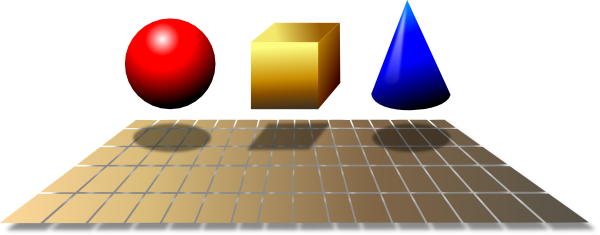Pull Down & Release to Refresh

# Definition of GeometryGeometry is the field of mathematics where points, lines, shapes and regions of space are studied.

Plane geometry is the geometry of shapes that can be fully contained in a plane (flat shapes) such as lines, circles and polygons.

Solid geometry is the geometry of 3-dimensional shapes such as spheres, cubes and polyhedra.

### Description

The aim of this dictionary is to provide definitions to common mathematical terms. Students learn a new math skill every week at school, sometimes just before they start a new skill, if they want to look at what a specific term means, this is where this dictionary will become handy and a go-to guide for a student.

### Audience

Year 1 to Year 12 students

### Learning Objectives

Learn common math terms starting with letter G

Author: Subject Coach
You must be logged in as Student to ask a Question.